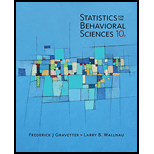# Sketch a graph showing the line for the equation Y = 2 X − 1 .On the same graph, show the line for Y = − X + 8 .### Statistics for The Behavioral Scie...

10th Edition
Frederick J Gravetter + 1 other
Publisher: Cengage Learning
ISBN: 9781305504912

#### Solutions

Chapter
Section### Statistics for The Behavioral Scie...

10th Edition
Frederick J Gravetter + 1 other
Publisher: Cengage Learning
ISBN: 9781305504912
Chapter 16, Problem 1P
Textbook Problem
78 views

## Sketch a graph showing the line for the equation Y = 2 X − 1 .On the same graph, show the line for Y = − X + 8 .

To determine

To graph:

Equation Y = 2X1 and Y = X + 8 in the same plot.

Solution:

We find the line Y=2X1 passing through points (2,3) and (1,1) and the line Y=X+8 passing through points (5,3) and (1,7). Both the lines are plotted on the same graph. We find that both the graphs intersect each other.

### Explanation of Solution

Steps:

• For equation Y=2X-1, we need to find two points that lie on this line
• So let X= 2, We get:
• Y=2x21 Y=41 Y=3
• Similarly, let X = 1, we get:
• Y=2x11 Y=21 Y=1
• Hence we get two points for equation Y=2X1 are: (2,3) and (1,1)
• Similarly for equation Y=X+8 we need to find two points
• For X = 5, we get:
• Y=5+8 Y=3
• And for X =1, we get:
• Y=1+8 Y=7
• Thus the two points for the equation Y=X+8 are: (5,3) (1,7)
• Plot x axis horizontally, and Y axis vertically and label both of them from 1 to 10Conclusion:

Thus with the help of equations, we can plug in some values of X as we want and predict the Y values. This gives us he points through with the line will pass. It helps us plot the line on the graph. If we use similar values of X it helps us to plot two different lines on the same graph.

#### The Solution to Your Study Problems

Bartleby provides explanations to thousands of textbook problems written by our experts, many with advanced degrees!

Get Started

Find more solutions based on key concepts
Convert the expressions in Exercises 6584 to power form. 8

Finite Mathematics and Applied Calculus (MindTap Course List)

If f(x)=0xx2sin(t2)dt, find f(x).

Single Variable Calculus: Early Transcendentals, Volume I

In Exercises 1-6. refer to the following figure, and determine the coordinates of each point and the quadrant i...

Applied Calculus for the Managerial, Life, and Social Sciences: A Brief Approach

Evaluate each expression: 5(37)+(8+4)3

Elementary Technical Mathematics

True or False: is a convergent series.

Study Guide for Stewart's Single Variable Calculus: Early Transcendentals, 8th

Finding a Derivative In Exercises 17-42, find the derivative of the function. h(x)=arccot6x

Calculus: Early Transcendental Functions (MindTap Course List)